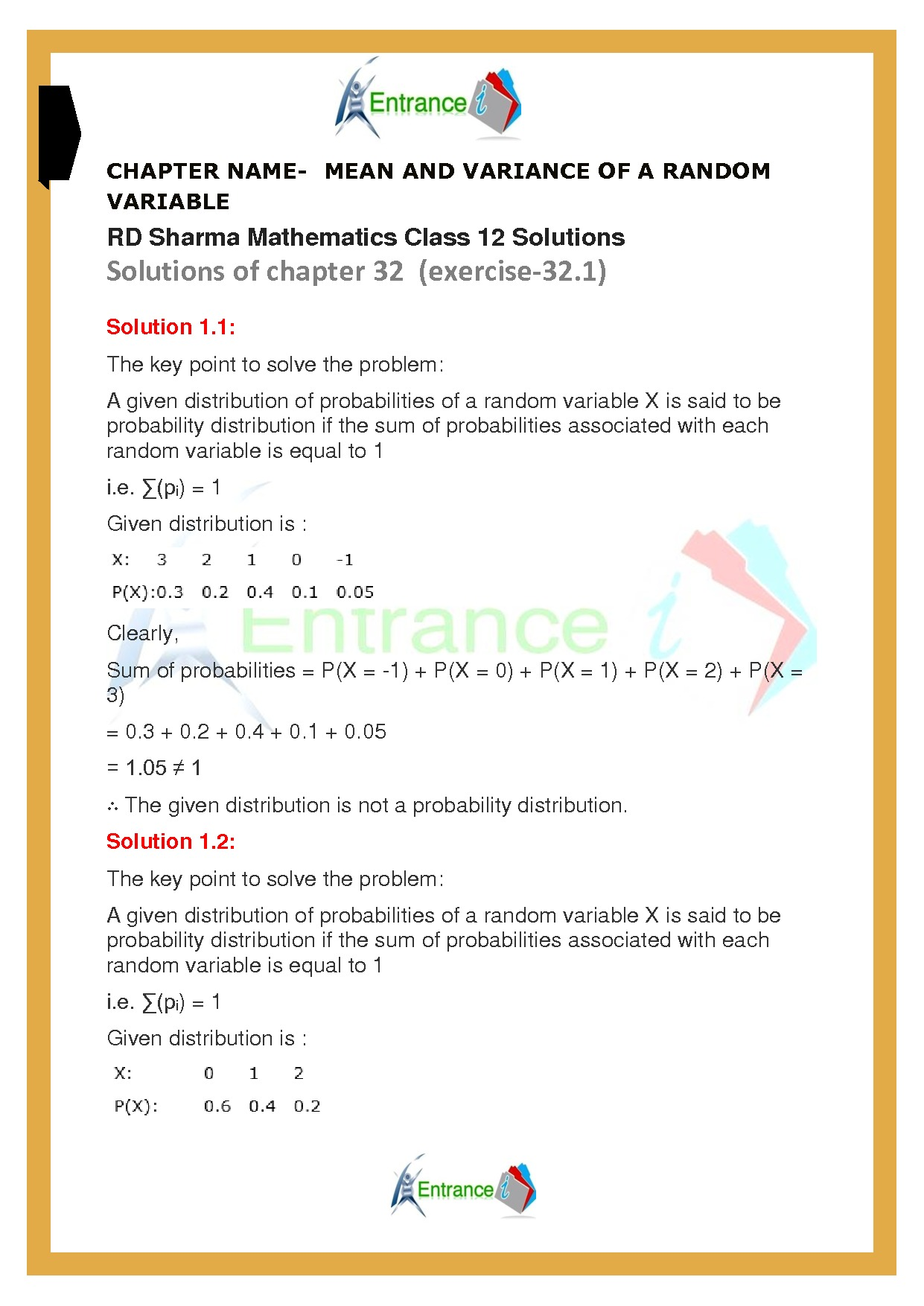# RD Sharma solutions for class 12 maths chapter-32 Exercise-32A

## RD Sharma solutions for class 12 maths chapter-32 Exercise-32A

Download chapter-32 Mean and variance of a random variable of RD Sharma text book for class 12 maths. All questions of exercise-32A are solved by expert’s faculty members.

Do read the maths formulas prepared by entrancei.

### Download free pdf of RD Sharma solutions for class 12 maths chapter-32 Exercise-32A